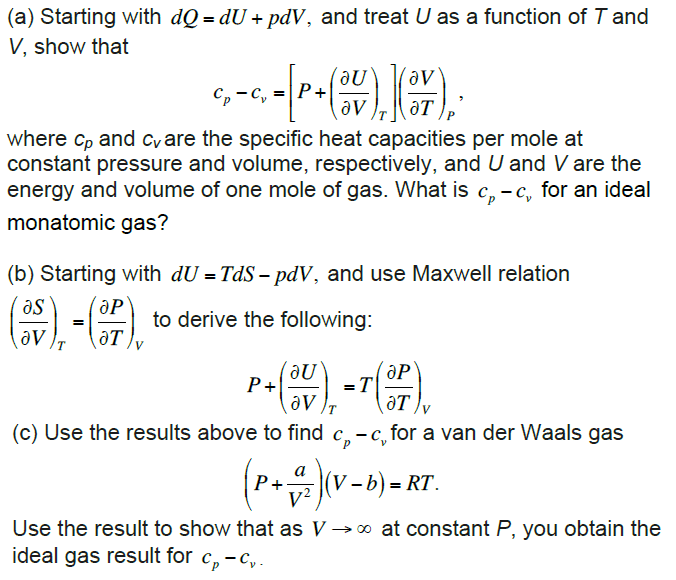# Maxwell relations

## Homework Statement

[/B]
I'm stuck on part c of the attached problem:## Homework Equations

$$C_P - C_V = \left[P + \left( \frac {∂U}{∂V} \right)_T \right]\left( \frac {∂V}{∂T} \right)_P$$

$$P + \left( \frac {∂U}{∂V} \right)_T = T \left( \frac {∂P}{∂T} \right)_V$$

$$\left(P + \frac {a}{V^2} \right)(V - b) = RT$$

## The Attempt at a Solution

I need to use the bottom two equations to find to find ##P + \left( \frac {∂U}{∂V} \right)_T ## and ##\left( \frac {∂V}{∂T} \right)_P## and plug these expressions in the top equation for CP - CV.

I've found $$\left (\frac {∂P}{∂T} \right)_V = \frac {R}{V - b}$$
$$∴ P + \left( \frac {∂U}{∂V} \right)_T = \frac {RT}{V - B}$$

But I'm having trouble finding ##\left (\frac {∂V}{∂T} \right)_P## because I can't seem to make V the subject of the Van der Waals gas expression.

#### Attachments

Homework Helper
Gold Member
Take the Van der Waal's equation and take a differential of both sides, using the product rule, (or just multiply out the left side), and treating ## P ## as a constant. You should get a couple of terms that are multiplied by ## dV ##. On the right side you will have ## R \, dT ##.

Last edited:
Take the Van der Waal's equation and take a differential of both sides, using the product rule, (or just multiply out the left side), and treating ## P ## as a constant. You should get a couple of terms that have are multiplied by ## dV ##. On the right side you will have ## R \, dT ##.

Oh I see. Thanks, I got it now :)

•